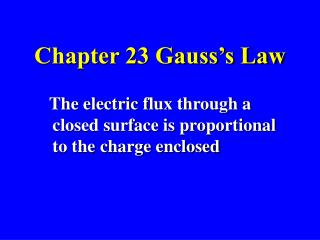DownloadDownload PresentationChapter 23 Gauss’s Law

# Chapter 23 Gauss’s Law

Télécharger la présentation## Chapter 23 Gauss’s Law

- - - - - - - - - - - - - - - - - - - - - - - - - - - E N D - - - - - - - - - - - - - - - - - - - - - - - - - - -
##### Presentation Transcript

1. Chapter 23 Gauss’s Law The electric flux through a closed surface is proportional to the charge enclosed

2. Carl Friedrich Gauss 1777-1855 Yes it’s the same guy that gave you the Gaussian distribution and … To give you some perspective he was born 50 years after Newton died (1642-1727). Predicted the time and place of the first asteroid CERES (Dec. 31, 1801). Had the unit of magnetic field named after him and of course had much to do with the development of mathematics Ceres t= 4.6 year , d=4.6 Au

3. FLUX

4. FLUX and the “dot-product”

5. Definition of Flux • The amount of field, material or other physical entity passing through a surface. • Surface area can be represented as vector defined normal to the surface it is describing • Defined by the equation:

6. Electric Flux • The amount of electric field passing through a surface area • The units of electric flux are N-m2/C

7. Gauss’ Law in E& M • Uses symmetry to determine E-field due to a charge distribution • Method: Considers a hypothetical surface enclosing some charge and calculates the E-field • The shape of that surface is “EVERYTHING”

8. KEY TO USING Gauss’s Law • The shape of the surrounding surface is one that MIMICS the symmetry of the charge distribution …..

9. Spherical Coordinates • Surface Integrals right (green)

10. The electric flux passing through a spherical surface surrounding a point charge !!! Note is shorthand for

11. The electric flux passing through a spherical surface surrounding a point charge

12. Gauss’s Law The previous example is true in general: The total flux passing through a closed surface is proportional to the charge enclosed within that surface. Note: The area vector points outward

13. The Gaussian Surface An imaginary closed surface created to enable the application of Gauss’s Law What is the total flux through each surface?

14. Solving problems with Gauss’s Law • Charge densities – It is convenient to define charge densities for linear, surface and volume charge distributions 2.Symmetry and coordinate systems – Choose a coordinate system that most nearly matches the symmetry of the charge distribution. For example, we chose spherical coordinates to determine the flux due to a point charge because of spherical symmetry.

15. Key!!! to solving problems with Gauss’s Law 3. Determining the Electric Field from Gauss’s Law - We will use Gauss’s Law to determine the electric field for problems for which the Electric Field can be shown to be constant in magnitude in direction for a particular Gaussian surface. Consider three examples: • The long straight line of charge • The infinite plane sheet of charge • The charged sphere.

16. Applied Gauss’ Law to Determine E-field in Cases where have • Spherical symmetry • Cylindrical symmetry • Planar symmetry

17. Example - Long straight line of charge Diagram (b) implies the problem has a cylindrical symmetry. Therefore cylindrical coordinates are appropriate. Three surfaces to consider. Upper and lower circular surfaces have normals parallel to the z axis which are perpendicular to the electric field, thus contribute zero to the flux. The integral to be evaluated is that of the cylinder of height l. The charge enclosed is ll.

18. Example - Long straight line of charge Since the field has radial symmetry, it is also constant at a fixed distance of r.

19. Results of other geometries Uniformly charged dielectric infinite plane sheet Uniformly charged dielectric (insulating) sphere Can you derive these results?

20. Gauss’ Law: Determining the E-field near the surface of a nonconducting (insulating) sheet

21. Conclusions Gauss’ LAW • Only the charge enclosed within a volume defined by a closed surface contributes to the net electric flux through the surface. • That net flux through the surface is proportional to the charge enclosed within the volume.

22. Conclusions 3. Gaussian surface is an imaginary closed surface necessary to solve a problem using Gauss’s Law 4. Gauss’s Law can be used to determine the electric field of a charge distribution if there is a high degree of symmetry

23. Conclusion: Gauss’ Law and Conductors 5. Applying Gauss’s Law to the interior of an electrostatically charged conductor we conclude that the electric field within the conductor is zero 6. Any Net charge on a conductor must reside on its surface

24. Shell Theorems: Conductors • A shell of uniform charge attracts or repels a charge particle that is outside the shell as though all charge is concentrated at the center. • If a charged particle is located inside such a shell, there is no electrostatic force on the particle from the shell

25. Electric Field at surface of conductor is perpendicular to the surface and proportional to the charge density at the surface Shown that the excess charge resides on the outer surface of conductor surface. Unless the surface is spherical the charge density s (chg. per unit area) varies However the E-field just outside of a conducting surface is easy to determine using Gauss’ Law